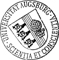## Asymptotically flat Fredholm bundles and the Novikov Conjecture

• The main kind of object considered in this thesis are ε-flat Fredholm bundles over simplicial complexes X. These consist of two Hilbert B-module bundles E^(0) and E^(1), equipped with connections with curvature of operator norm bounded by ε and an operator F: E^(0)->E^(1) which restricts to Fredholm operators on the fibres of E^(0) and E^(1), and which commutes with parallel transport up to compact operators. We consider ε-flat Fredholm bundles in the limit ε->0. Our main result is a kind of index theorem which computes the index of these almost flat Fredholm bundles in terms of so-called asymptotic Fredholm representations of the fundamental group. These asymptotic Fredholm representations only depend on the Fredholm operator on the fibre over a basepoint of the base space X and on the parallel transport along a finite set of curves in the geometric realization of X. We make essential use of Thomsen's D-theory, which is a discrete variant of the E-theory of Connes and Higson. AsThe main kind of object considered in this thesis are ε-flat Fredholm bundles over simplicial complexes X. These consist of two Hilbert B-module bundles E^(0) and E^(1), equipped with connections with curvature of operator norm bounded by ε and an operator F: E^(0)->E^(1) which restricts to Fredholm operators on the fibres of E^(0) and E^(1), and which commutes with parallel transport up to compact operators. We consider ε-flat Fredholm bundles in the limit ε->0. Our main result is a kind of index theorem which computes the index of these almost flat Fredholm bundles in terms of so-called asymptotic Fredholm representations of the fundamental group. These asymptotic Fredholm representations only depend on the Fredholm operator on the fibre over a basepoint of the base space X and on the parallel transport along a finite set of curves in the geometric realization of X. We make essential use of Thomsen's D-theory, which is a discrete variant of the E-theory of Connes and Higson. As an application of our index theorem, we show that a K-homology class which is detected by indices of ε-flat Fredholm bundles for arbitrarily small ε>0 is mapped to a nonzero class under the maximal assembly map. We show how to use this statement to give a new proof for a special case of Yu's result that the Strong Novikov Conjecture holds for groups with finita asymptotic dimension.• Hunger_Diss.pdfAuthor: Benedikt HungerORCiD urn:nbn:de:bvb:384-opus4-832501 https://opus.bibliothek.uni-augsburg.de/opus4/83250 Bernhard Hanke Doctoral Thesis English 2020 Universität Augsburg Universität Augsburg, Mathematisch-Naturwissenschaftlich-Technische Fakultät 2020/11/24 2021/04/06 Novikov-Vermutung; Fredholm-Operator; Bündel ; Hilbert-Modul; Operatoralgebra Topologieoptimierung; Homogenisierung ; Zerstörungsfreie Werkstoffprüfung; Mathematische Modellierung; Computersimulation; Mathematische Physik Mathematisch-Naturwissenschaftlich-Technische Fakultät Mathematisch-Naturwissenschaftlich-Technische Fakultät / Institut für Mathematik Mathematisch-Naturwissenschaftlich-Technische Fakultät / Institut für Mathematik / Lehrstuhl für Differentialgeometrie 5 Naturwissenschaften und Mathematik / 51 Mathematik / 510 MathematikDeutsches Urheberrecht